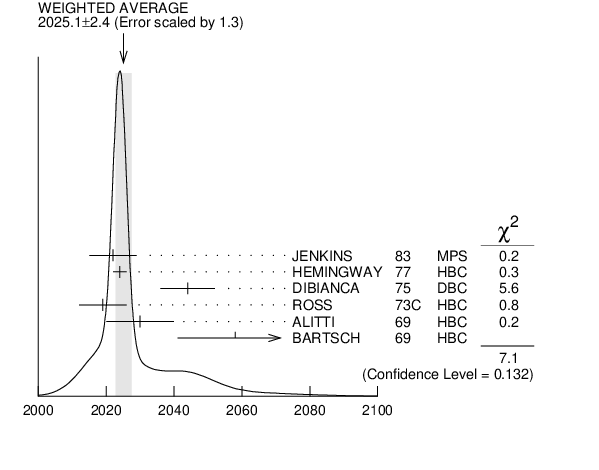# ${{\boldsymbol \Xi}{(2030)}}$ MASS INSPIRE search

VALUE (MeV) EVTS DOCUMENT ID TECN CHG  COMMENT
$\bf{ 2025 \pm5}$ OUR ESTIMATE
$\bf{ 2025.1 \pm2.4}$ OUR AVERAGE  Error includes scale factor of 1.3.
$2022$ $\pm7$
 1983
MPS - ${{\mathit K}^{-}}$ ${{\mathit p}}$ $\rightarrow$ ${{\mathit K}^{+}}$ MM
$2024$ $\pm2$ 200
 1977
HBC - ${{\mathit K}^{-}}{{\mathit p}}$ 4.2 ${\mathrm {GeV/}}\mathit c$
$2044$ $\pm8$
 1975
DBC -0 ${{\mathit \Xi}}{{\mathit \pi}}{{\mathit \pi}}$ , ${{\mathit \Xi}^{*}}{{\mathit \pi}}$
$2019$ $\pm7$ 15
 1973 C
HBC -0 ${{\mathit \Sigma}}{{\overline{\mathit K}}}$
$2030$ $\pm10$ 42
 1969
HBC - ${{\mathit K}^{-}}{{\mathit p}}$ 3.9$-$5 ${\mathrm {GeV/}}\mathit c$
$2058$ $\pm17$ 40
 1969
HBC -0 ${{\mathit K}^{-}}{{\mathit p}}$ 10 ${\mathrm {GeV/}}\mathit c$${{\mathit \Xi}{(2030)}}$ mass (MeV)
References:
 JENKINS 1983
PRL 51 951 Existence of ${{\mathit \Xi}}$ Resonances above 2 GeV
 HEMINGWAY 1977
PL 68B 197 ${{\mathit \Xi}{(2030)}^{-}}$ Production in ${{\mathit K}^{-}}{{\mathit p}}$ Reactions at 4.2 ${\mathrm {GeV/}}\mathit c$
 DIBIANCA 1975
NP B98 137 Study of ${{\mathit \Xi}^{-}}$ and ${{\mathit \Omega}^{-}}$ Production from ${{\mathit K}^{-}}{{\mathit n}}$ and ${{\mathit K}^{-}}{{\mathit p}}$ Interactions at 4.93 ${\mathrm {GeV/}}\mathit c$
 ROSS 1973C
Purdue Conf. 345 Production of Strangeness -2 Baryons in the Mass Region 1600 to 2100 ${\mathrm {MeV}}/\mathit c$2 in ${{\mathit K}^{-}}{{\mathit p}}$ Interactions at 3.1, 3.3, and 3.6 ${\mathrm {GeV/}}\mathit c$
 ALITTI 1969
PRL 22 79 Strangeness S = -2 Baryon Resonance
 BARTSCH 1969
PL 28B 439 Evidence for a New ${{\mathit \Xi}}$ Resonance at 2500 MeV in 10 ${\mathrm {GeV/}}\mathit c$ ${{\mathit K}^{-}}{{\mathit p}}$ Interactions